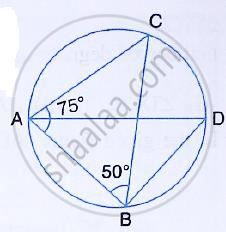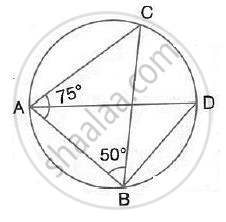Share

Given: ∠Cab = 75° and ∠Cba = 50°. Find the Value of ∠Dab + ∠Abd. - ICSE Class 10 - Mathematics

ConceptChord Properties - Chords Equidistant from the Center Are Equal (Without Proof)

Question

Given: ∠CAB = 75° and ∠CBA = 50°. Find the value of ∠DAB + ∠ABD.SolutionIn ΔABC, ∠CBA = 50° ,∠CAB = 75°
∠ACB =180° - (∠CBA+CAB)
= 180° - (50° + 75° )
= 180° -125°
= 55°
But ∠ADB = ∠ACB = 55°
(Angle subtended by the same chord on the circle are equal)
Now consider ΔABD,
∠DAB + ∠ABD + ∠ADB =180°
⇒ ∠DAB +∠ABD+ 55° = 180°
⇒ ∠DAB +∠ABD =180° - 55°
⇒ ∠DAB + ∠ABD + 125°

Is there an error in this question or solution?

Video TutorialsVIEW ALL 

Solution Given: ∠Cab = 75° and ∠Cba = 50°. Find the Value of ∠Dab + ∠Abd. Concept: Chord Properties - Chords Equidistant from the Center Are Equal (Without Proof).
S